# Check whether the given node is in the path between the nodes U and V

Given three vertices U, V and R of a binary tree, the task is to check whether R lies in the path between U and V. If it is not present in the path then print No otherwise print Yes.

Examples:

Input: U = 4, V = 6, R = 2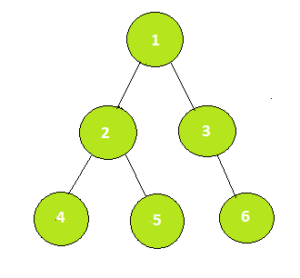Output: Yes
Path 4 -> 2 -> 1 -> 3 -> 6 contains 2

Input: U = 4, V = 6, R = 5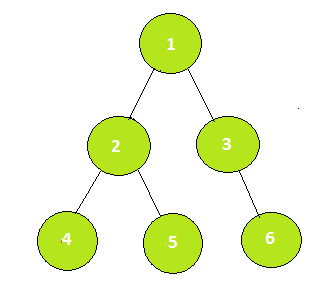Output: No
Path 4 -> 2 -> 1 -> 3 -> 6 does not contain 5

## Recommended: Please try your approach on {IDE} first, before moving on to the solution.

Approach: The idea is to use Lowest Common Ancestor of two nodes. There are following cases for R to exist in the path between U and V:

1. R is the lowest common ancestor of U and V.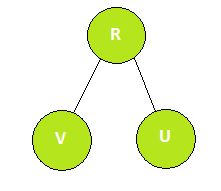2. R is in the left subtree of the lowest common ancestor of U and V and is above V.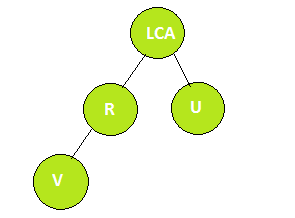3. R is in the right subtree of the lowest common ancestor of U and V and is above U.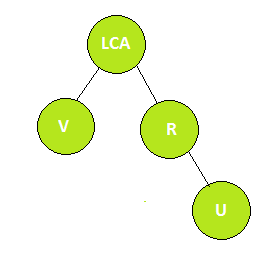To know more about the lowest commom ancestor, read the post here.

Below is the implementation of the above approach:

## Java

 `// Java implementation of the approach ` `import` `java.util.*; ` ` `  `class` `GfG { ` ` `  `    ``// Table for storing 2^ith parent ` `    ``private` `static` `int` `table[][]; ` ` `  `    ``// Variable to store the height of the tree ` `    ``private` `static` `int` `height; ` ` `  `    ``// Graph ` `    ``private` `static` `ArrayList > Graph; ` ` `  `    ``// Arrays to mark start and end time for a node ` `    ``private` `static` `int` `timeIn[]; ` `    ``private` `static` `int` `timeOut[]; ` ` `  `    ``// Timer ` `    ``private` `static` `int` `time; ` ` `  `    ``// Private constructor for initializing ` `    ``// the global variables ` `    ``private` `GfG(``int` `n) ` `    ``{ ` ` `  `        ``// log(n) with base 2 ` `        ``height = (``int``)Math.ceil(Math.log10(n) / Math.log10(``2``)); ` `        ``table = ``new` `int``[n + ``1``][height + ``1``]; ` ` `  `        ``// Fill the graph with empty lists ` `        ``Graph = ``new` `ArrayList >(); ` `        ``for` `(``int` `i = ``0``; i <= n; i++) ` `            ``Graph.add(``new` `ArrayList()); ` `        ``timeIn = ``new` `int``[n + ``1``]; ` `        ``timeOut = ``new` `int``[n + ``1``]; ` `        ``time = ``0``; ` `    ``} ` ` `  `    ``// Filling with -1 as initial ` `    ``private` `static` `void` `preprocessing(``int` `n) ` `    ``{ ` `        ``for` `(``int` `i = ``0``; i < n + ``1``; i++) { ` `            ``Arrays.fill(table[i], -``1``); ` `        ``} ` `    ``} ` ` `  `    ``// Dfs for pre-processing sparse table and ` `    ``// calculating start and end time ` `    ``private` `static` `void` `dfs(``int` `s, ``int` `p) ` `    ``{ ` `        ``// Parent at 1 node distance is always ` `        ``// it's direct parent ` `        ``table[s][``0``] = p; ` ` `  `        ``// Start time noted ` `        ``timeIn[s] = ++time; ` ` `  `        ``// Filling sparse table recursively ` `        ``for` `(``int` `i = ``1``; i <= height; i++) ` `            ``table[s][i] = table[table[s][i - ``1``]][i - ``1``]; ` ` `  `        ``// Traversing children of source ` `        ``for` `(``int` `child : Graph.get(s)) { ` `            ``if` `(child == p) ` `                ``continue``; ` `            ``dfs(child, s); ` `        ``} ` ` `  `        ``// End time noted ` `        ``timeOut[s] = ++time; ` `    ``} ` ` `  `    ``// Helper function to check lowest common Ancestor ` `    ``private` `static` `boolean` `check(``int` `u, ``int` `v) ` `    ``{ ` `        ``return` `timeIn[u] <= timeIn[v] && timeOut[u] >= timeOut[v]; ` `    ``} ` ` `  `    ``// Function to return Lowest Common Ancestor of U and V ` `    ``private` `static` `int` `lowestCommonAncestor(``int` `U, ``int` `V) ` `    ``{ ` `        ``if` `(check(U, V)) ` `            ``return` `U; ` ` `  `        ``if` `(check(V, U)) ` `            ``return` `V; ` ` `  `        ``for` `(``int` `i = height; i >= ``0``; i--) { ` `            ``if` `(!check(table[U][i], V)) ` `                ``U = table[U][i]; ` `        ``} ` ` `  `        ``return` `table[U][``0``]; ` `    ``} ` ` `  `    ``// Function that return true if R ` `    ``// exists on the path between U ` `    ``// and V in the given tree ` `    ``private` `static` `boolean` `isPresent(``int` `U, ``int` `V, ``int` `R) ` `    ``{ ` ` `  `        ``// Dfs ` `        ``dfs(``1``, ``1``); ` ` `  `        ``// Calculating LCA between U and V ` `        ``int` `LCA = lowestCommonAncestor(U, V); ` ` `  `        ``// Calculating LCA between U and R ` `        ``int` `LCA_1 = lowestCommonAncestor(U, R); ` ` `  `        ``// Calculating LCA between U and V ` `        ``int` `LCA_2 = lowestCommonAncestor(V, R); ` ` `  `        ``if` `(LCA == R || (LCA_1 == LCA && LCA_2 == R) ` `            ``|| (LCA_2 == LCA && LCA_1 == R)) { ` `            ``return` `true``; ` `        ``} ` `        ``return` `false``; ` `    ``} ` ` `  `    ``// Driver code ` `    ``public` `static` `void` `main(String args[]) ` `    ``{ ` `        ``// Number of vertices ` `        ``int` `n = ``6``; ` `        ``GfG obj = ``new` `GfG(n); ` ` `  `        ``// Create the graph ` `        ``preprocessing(n); ` `        ``Graph.get(``1``).add(``2``); ` `        ``Graph.get(``2``).add(``1``); ` `        ``Graph.get(``1``).add(``3``); ` `        ``Graph.get(``3``).add(``1``); ` `        ``Graph.get(``2``).add(``4``); ` `        ``Graph.get(``4``).add(``2``); ` `        ``Graph.get(``2``).add(``5``); ` `        ``Graph.get(``5``).add(``2``); ` `        ``Graph.get(``3``).add(``6``); ` `        ``Graph.get(``6``).add(``3``); ` ` `  `        ``int` `U = ``4``, V = ``6``, R = ``2``; ` `        ``if` `(isPresent(U, V, R)) ` `            ``System.out.print(``"Yes"``); ` `        ``else` `            ``System.out.print(``"No"``); ` `    ``} ` `} `

## C#

 `// C# implementation of the approach ` `using` `System; ` `using` `System.Collections.Generic; ` ` `  `class` `GfG  ` `{ ` ` `  `    ``// Table for storing 2^ith parent ` `    ``private` `static` `int` `[,]table; ` ` `  `    ``// Variable to store the height of the tree ` `    ``private` `static` `int` `height; ` ` `  `    ``// Graph ` `    ``private` `static` `List > Graph; ` ` `  `    ``// Arrays to mark start and end time for a node ` `    ``private` `static` `int` `[]timeIn; ` `    ``private` `static` `int` `[]timeOut; ` ` `  `    ``// Timer ` `    ``private` `static` `int` `time; ` ` `  `    ``// Private constructor for initializing ` `    ``// the global variables ` `    ``private` `GfG(``int` `n) ` `    ``{ ` ` `  `        ``// log(n) with base 2 ` `        ``height = (``int``)Math.Ceiling(Math.Log10(n) / Math.Log10(2)); ` `        ``table = ``new` `int``[n + 1, height + 1]; ` ` `  `        ``// Fill the graph with empty lists ` `        ``Graph = ``new` `List >(); ` `        ``for` `(``int` `i = 0; i <= n; i++) ` `            ``Graph.Add(``new` `List<``int``>()); ` `        ``timeIn = ``new` `int``[n + 1]; ` `        ``timeOut = ``new` `int``[n + 1]; ` `        ``time = 0; ` `    ``} ` ` `  `    ``// Filling with -1 as initial ` `    ``private` `static` `void` `preprocessing(``int` `n) ` `    ``{ ` `        ``for` `(``int` `i = 0; i < n + 1; i++)  ` `        ``{ ` `            ``for``(``int` `j = 0; j < height + 1; j++) ` `                ``table[i, j] = -1; ` `        ``} ` `    ``} ` ` `  `    ``// Dfs for pre-processing sparse table and ` `    ``// calculating start and end time ` `    ``private` `static` `void` `dfs(``int` `s, ``int` `p) ` `    ``{ ` `        ``// Parent at 1 node distance is always ` `        ``// it's direct parent ` `        ``table[s, 0] = p; ` ` `  `        ``// Start time noted ` `        ``timeIn[s] = ++time; ` ` `  `        ``// Filling sparse table recursively ` `        ``for` `(``int` `i = 1; i <= height; i++) ` `            ``table[s, i] = table[table[s, i - 1], i - 1]; ` ` `  `        ``// Traversing children of source ` `        ``foreach` `(``int` `child ``in` `Graph[s]) ` `        ``{ ` `            ``if` `(child == p) ` `                ``continue``; ` `            ``dfs(child, s); ` `        ``} ` ` `  `        ``// End time noted ` `        ``timeOut[s] = ++time; ` `    ``} ` ` `  `    ``// Helper function to check lowest common Ancestor ` `    ``private` `static` `bool` `check(``int` `u, ``int` `v) ` `    ``{ ` `        ``return` `timeIn[u] <= timeIn[v] && timeOut[u] >= timeOut[v]; ` `    ``} ` ` `  `    ``// Function to return Lowest Common Ancestor of U and V ` `    ``private` `static` `int` `lowestCommonAncestor(``int` `U, ``int` `V) ` `    ``{ ` `        ``if` `(check(U, V)) ` `            ``return` `U; ` ` `  `        ``if` `(check(V, U)) ` `            ``return` `V; ` ` `  `        ``for` `(``int` `i = height; i >= 0; i--)  ` `        ``{ ` `            ``if` `(!check(table[U, i], V)) ` `                ``U = table[U, i]; ` `        ``} ` ` `  `        ``return` `table[U, 0]; ` `    ``} ` ` `  `    ``// Function that return true if R ` `    ``// exists on the path between U ` `    ``// and V in the given tree ` `    ``private` `static` `bool` `isPresent(``int` `U, ``int` `V, ``int` `R) ` `    ``{ ` ` `  `        ``// Dfs ` `        ``dfs(1, 1); ` ` `  `        ``// Calculating LCA between U and V ` `        ``int` `LCA = lowestCommonAncestor(U, V); ` ` `  `        ``// Calculating LCA between U and R ` `        ``int` `LCA_1 = lowestCommonAncestor(U, R); ` ` `  `        ``// Calculating LCA between U and V ` `        ``int` `LCA_2 = lowestCommonAncestor(V, R); ` ` `  `        ``if` `(LCA == R || (LCA_1 == LCA && LCA_2 == R) ` `            ``|| (LCA_2 == LCA && LCA_1 == R))  ` `        ``{ ` `            ``return` `true``; ` `        ``} ` `        ``return` `false``; ` `    ``} ` ` `  `    ``// Driver code ` `    ``public` `static` `void` `Main(String []args) ` `    ``{ ` `        ``// Number of vertices ` `        ``int` `n = 6; ` `        ``GfG obj = ``new` `GfG(n); ` ` `  `        ``// Create the graph ` `        ``preprocessing(n); ` `        ``Graph.Add(2); ` `        ``Graph.Add(1); ` `        ``Graph.Add(3); ` `        ``Graph.Add(1); ` `        ``Graph.Add(4); ` `        ``Graph.Add(2); ` `        ``Graph.Add(5); ` `        ``Graph.Add(2); ` `        ``Graph.Add(6); ` `        ``Graph.Add(3); ` ` `  `        ``int` `U = 4, V = 6, R = 2; ` `        ``if` `(isPresent(U, V, R)) ` `            ``Console.Write(``"Yes"``); ` `        ``else` `            ``Console.Write(``"No"``); ` `    ``} ` `} ` ` `  `// This code is contributed by PrinciRaj1992 `

Output:

```Yes
```

Time Complexity: O(NlogN) for pre-processing and logN for finding the lowest common ancestor.

Attention reader! Don’t stop learning now. Get hold of all the important DSA concepts with the DSA Self Paced Course at a student-friendly price and become industry ready.

My Personal Notes arrow_drop_upCheck out this Author's contributed articles.

If you like GeeksforGeeks and would like to contribute, you can also write an article using contribute.geeksforgeeks.org or mail your article to contribute@geeksforgeeks.org. See your article appearing on the GeeksforGeeks main page and help other Geeks.

Please Improve this article if you find anything incorrect by clicking on the "Improve Article" button below.

Improved By : princiraj1992